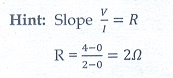Home | | Physics 12th Std | Current Electricity: Multiple Choice Questions with Answer

# Current Electricity: Multiple Choice Questions with Answer

Physics : Current Electricity: Multiple choice questions with Answers, Solution and Explanation, Physics Book Back 1 mark questions with answers, solution

## Multiple Choice Questions

1. The following graph shows current versus voltage values of some unknown conductor. What is the resistance of this conductor?(a) 2 ohm

(b) 4 ohm

(c) 8 ohm

(d)1 ohm2. A wire of resistance 2 ohms per meter is bent to form a circle of radius 1m. The equivalent resistance between its two diametrically opposite points, A and B as shown in the figure is(a) ŽĆ ╬®

(b)ŽĆ/2╬®

(c) 2ŽĆ╬®

(d)ŽĆ/4╬®3. A toaster operating at 240 V has a resistance of 120 ╬®. The power is

a) 400 W

b) 2 W

c) 480 W

d) 240 W4. A carbon resistor of (47 ┬▒ 4.7 ) k ╬® to be marked with rings of different colours for its identification. The colour code sequence will be

a.     Yellow ŌĆō Green ŌĆō Violet ŌĆō Gold

b.    Yellow ŌĆō Violet ŌĆō Orange ŌĆō Silver

c.      Violet ŌĆō Yellow ŌĆō Orange ŌĆō Silver

d.     Green ŌĆō Orange ŌĆō Violet - Gold5.What is the value of resistance of the following resistor?(a)100 k ╬®

(b)10 k ╬®

(c) 1k ╬®

(d)1000 k ╬®6. Two wires of A and B with circular cross section made up of the same material with equal lengths. Suppose RA = 3 RB, then what is the ratio of radius of wire A to that of B?

(a) 3

(b) ŌłÜ3

(c) 1/ ŌłÜ3

(d)1/37. A wire connected to a power supply of 230 V has power dissipation P1. Suppose the wire is cut into two equal pieces and connected parallel to the same power supply. In this case power dissipation is P2. The ratio P2 / P1 is

(a) 1

(b) 2

(c) 3

(d) 48. In India electricity is supplied for domestic use at 220 V. It is supplied at 110 V in USA. If the resistance of a 60W bulb for use in India is R, the resistance of a 60W bulb for use in USA will be

(a) R

(b) 2R

(c)R/4

(d)R/29.In a large building, there are 15 bulbs of 40W, 5 bulbs of 100W, 5 fans of 80W and 1 heater of 1kW are connected. The voltage of electric mains is 220V. The minimum capacity of the main fuse of the building will be

(a) 14 A

(b) 8 A

(c) 10 A

(d) 12 A10. There is a current of 1.0 A in the circuit shown below. What is the resistance of P ?a) 1.5 ╬®

b) 2.5 ╬®

c) 3.5 ╬®

d) 4.5 ╬®11.  What is the current out of the battery?a) 1A

b) 2A

c) 3A

d) 4A12. The temperature coefficient of resistance of a wire is 0.00125 per ┬░C. At 300 K, its resistance is 1ŌĆå╬®. The resistance of the wire will be 2ŌĆå╬® at

a) 1154 K

b) 1100 K

c) 1400 K

d) 1127 K13. The internal resistance of a 2.1 V cell which gives a current of 0.2 A through a resistance of 10 ╬® is

a) 0.2 ╬®

b) 0.5 ╬®

c) 0.8 ╬®

d) 1.0 ╬®14. A piece of copper and another of germanium are cooled from room temperature to 80 K. The resistance of

a.     each of them increases

b.     each of them decreases

c.      copper increases and germanium decreases

d.    copper decreases and germanium increases15.In JouleŌĆÖs heating law, when I and t are constant, if the H is taken along the y axis and I2 along the x axis, the graph is

a) straight line

b) parabola

c) circle

d) ellipseTags : Physics , 12th Physics : Current Electricity
Study Material, Lecturing Notes, Assignment, Reference, Wiki description explanation, brief detail
12th Physics : Current Electricity : Current Electricity: Multiple Choice Questions with Answer | Physics